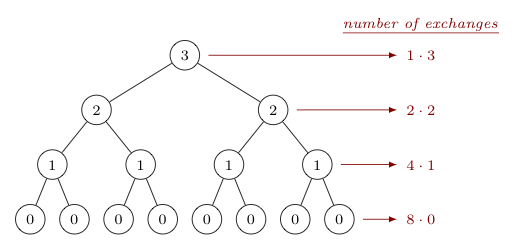## Heapsort Analysis

To find the comparison and exchange costs for the heapsort process, we consider the two phases (heapification of the original array and repeated removal of maximum elements) separately. The second phase proves easier to analyze, so we start with it.

Note that removing the maximum element from a heap of $n$ items involves $O(\log_2 n)$ exchanges as this is the height of a binary tree of $n$ elements, and thus the maximum number of sinks that will happen to the item that replaces the root upon dequeuement.

As such, the exchange cost of removing $n$ elements is $O(n \log_2 n)$.

In sinking, recall that we must compare nodes with both of their children, while exchanges involve only one of these. As such, the comparison cost is twice that of the exchange cost. However, being only a constant multiple of the exchange cost, it is again $O(n \log_2 n)$.

Now let us consider the exchange cost of the first part of the heapsort algorithm -- the "heapification" of the array to be sorted.

(Be aware, there's a wee little bit of calculus ahead..)

Let's suppose the tree in question has height $h$. Then in the worst case, we need to heapify $2^{h+1}-1$ items. Suppose -- also in the worst case -- that at every level, every node sinks to the bottom-most level.

That means that the $2^h$ leaves didn't fall any levels (requiring $0$ exchanges), the $2^{h-1}$ nodes directly above these all fell $1$ level (requiring $2^{h-1}$ exchanges total), the $2^{h-2}$ nodes on the level above those nodes fell $2$ levels (requiring $2^{h-2} \cdot 2$ exchanges total), and so on. As an example, the tree below shows the number of exchanges required in the worst case when the height of the tree is $h=3$.To calculate $C(n)$ for exchanges made during heapification in the worst case, we thus first replace $n$ with its equivalent expression in terms of $h$, and then find (in terms of $h$) the sum of exchanges associated with each level, from the bottom-most level to the root. Writing the expression in summation notation will be convenient, as well.

$$\begin{array}{rcl} C(n) &=& C(2^h-1)\\ &=& 2^h \cdot 0 + 2^{h-1} \cdot 1 + 2^{h-2} \cdot 2 + 2^{h-3} \cdot 3 + \cdots + 2^{h-h} \cdot h\\ &=& \displaystyle{\sum_{i=0}^h 2^{h-i} i}\\ &=& \displaystyle{2^h \sum_{i=0}^h \frac{i}{2^i}}\\ \end{array}$$

There is a clever trick for dealing with the last sum above, which may not be immediately familiar. First recall the sum of an infinite geometric series for any constant ratio $x \lt 1$:

$$\sum_{i=0}^{\infty} x^i = \frac{1}{1-x}$$

Taking a derivative of both sides with respect to $x$, and then multiplying by $x$, we have

$$\sum_{i=0}^{\infty} i x^{i-1} = \frac{1}{(1-x)^2} \quad \textrm{ and then } \quad \sum_{i=0}^{\infty} i x^i = \frac{x}{(1-x)^2}$$

Now substitute $x = 1/2$ and we have

$$\sum_{i=0}^{\infty} \frac{i}{2^i} = \frac{1/2}{(1-(1/2))^2} = \frac{1/2}{1/4} = 2$$

Of course, we are interested in the sum from $i=0$ to $i=h$ instead -- which will be smaller. As such,

$$C(n) \lt 2^h \cdot 2 = 2^{h+1}$$

But recall $n = 2^{h+1} - 1$, so the exchange cost $C(n)$ in the worst case is approximately $n$.

Remembering that earlier above we said the comparison cost was twice the exchange cost, it must be that in the worst case the comparison cost is approximately $2n$.

Of course, both of these are smaller than the bound $O(n \log_2 n)$ on the cost of the second phase, found at the beginning of our discussion.

As a result, the overall exchange and comparison costs for heapsort is $O(n \ln n)$.